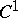# Trough

(diff) ← Older revision | Latest revision (diff) | Newer revision → (diff)
A doubly-connected surfaceof annular type, containing a planar closed curvethe planeof which is tangent toat all points of. Alongthe Gaussian curvatureofvanishes. If, in these circumstances,dividesinto two parts on each of whichis of constant sign, then the corresponding parts ofare called the positive and negative semi-trough. An example of a trough is a narrow band of a torus along one of its closed parabolic parallels.
A trough occupies an intermediate position between the objects of "global" and "local" geometry, since, because it contains a specific closed curve, it cannot be arbitrarily small, while its dimensions in directions transversal tomay be arbitrary small. Interest in the study of troughs is aroused by the fact that a sufficiently narrow band of surfaces of alternating curvature along a closed parabolic line is often a trough, and for this reason, knowing the properties of troughs under various deformations sometimes enables one to obtain information on the corresponding properties of the surface "in the large" .
The most detailed research has been carried out into the so-called planar troughs (for which the curveis convex, while the troughitself is positioned along one side of, with which it has a contact of the first order) and troughs of rotation (whenis a parallel of the surface of rotation). For analytic planar troughs, their rigidity relative to analytic infinitesimal deformations of the second order has been proved. For troughs of rotation, the study of their infinitesimal deformations of the first and second orders has been extended to the regularity class. From the point of view of differential equations, research into troughs reduces to the study of equations of mixed type.